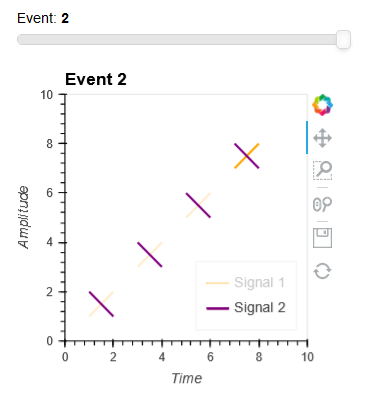# Strange legend behavior(/bug) for same labeled plots in an Overlay and DynamicMap?

Dear holoviews developers and community,

thanks a lot for this awesome package. I have a question regarding legends of similarly labeled plots within an overlay using a stream in a DynamicMap. I noticed that the legend breaks if the number of plotted object changes. E.g. I have two type of signals where the number of signals can change per event. Please also see the following MWE and attached images.

#Define some dummy data with different number of signals per event:
x_test_data_1 = [[[1,2], [3, 4], [5, 6]], #Event 1
[[1,2], [3, 4]], #Event 2
[[1,2], [3, 4], [5, 6], [7, 8]], #Event 3
]
y_test_data_1 = [[[1,2], [3, 4], [5, 6]], #Event 1
[[1,2], [3, 4]], #Event 2
[[1,2], [3, 4], [5, 6], [7, 8]] #Event 3
]

x_test_data_2 = x_test_data_1
y_test_data_2 = [[[2, 1], [4, 3], [6, 5]], #Event 1
[[2,1], [4, 3]], #Event 2
[[2,1], [4, 3], [6, 5], [8, 7]] #Event 3
]

# Define dummy callback as used by application:

def test_callback(index):
x1, y1 = x_test_data_1[index], y_test_data_1[index]
x2, y2 = x_test_data_2[index], y_test_data_2[index]

``````signal_1_plots = []
for x,y in zip(x1, y1):
signal_1 = hv.Curve((x, y),
kdims=hv.Dimension('x', label='Time'),
vdims=[hv.Dimension('y', label='Amplitude'),],
label='Signal 1', group=f'Event {index}').opts(color='orange')
signal_1_plots.append(signal_1)

signal_2_plots = []
for x,y in zip(x2, y2):
signal_2 = hv.Curve((x, y),
kdims=hv.Dimension('x', label='Time'),
vdims=[hv.Dimension('y', label='Amplitude'),],
label='Signal 2',
group=f'Event {index}').opts(color='purple')
signal_2_plots.append(signal_2)

return hv.Overlay(signal_1_plots + signal_2_plots).opts(xlim=(0, 10),
ylim=(0, 10),
legend_position='bottom_right')
``````

# Create DynamicMap

test_slider = pn.widgets.IntSlider(start=0, end=len(x_test_data_1)-1, value=0, name=‘Event’)
plot = hv.DynamicMap(test_callback, streams={‘index’: test_slider})
pn.Column(test_slider, plot)

Plotting less or more objects breaks the legend (sorry I wanted to attache more images but I am not allowed as a new user):I am not sure if this can be considered a bug or an unwanted side effect due to a mistake from my side. I am open for any suggestion. Thanks a lot.

Maybe some more context about the application:
I am working on an experiment in antiparticle physics and I am currently trying to replace our current interactive event display which is based on bokeh with a new one using holoviews. In general our detector produces two types of different signals which can occur within a single event. The number of signals per type per event can vary.

FYI: I found a similar behavior for hv.Text, see MWE below. While the span is updated correctly the text of the third signal is plotted at the position of the second event when plotting the third one.To me it looks like a bug. At least I do not understand why this should be the expected behavior.

x_test_data_1 = [[[1,2], [3, 4]], #Event 1 with 2 signals
[[1,2], [3, 4], [5, 6]], #Event 2 with 3 signals
[[1,2], [3, 4], [7, 8]], #Event 3 with 3 signals
]

def _dummy_text_span_callback(event_index):
times = x_test_data_1[event_index]

``````shaded_regions = []
for ind, (start,end) in enumerate(times):
span = hv.VSpan(start, end).opts(color='gray', alpha=0.4)
text = hv.Text(start, 0, rotation=90,
text=f'Signal{ind}', valign='bottom', halign='left')